# Physics Homework Help With Rocket Propulsion

1. Home
2. Physics
3. Impulse And Momentum
4. System Of Variable Mass
5. Rocket Propulsion

Rocket of mass m0 is launched vertically from the ground. The constant rate at which the gas is burnt is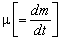and the exhaust velocity with respect to rocket is v. Let us calculate the acceleration of the rocket and its velocity of the rocket when its mass is m. Assume that acceleration due to gravity is constant during the motion in question.

Let m be the mass of the rocket moving with velocity V at some instant t.

Thrust or rocketvdm/dt = µv(­)

Now,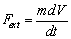Þ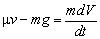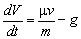.. (i)

Þ Now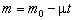equation (i)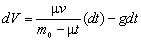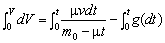For integration put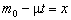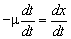Þ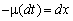Þ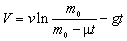### Homework Help For Rocket Propulsion

assignmenthelp.net provides best Online Assignment Help service in Physics for all standards. Our Tutor provide their high quality and optimized Tutorial help to fulfill all kind of need of Students.